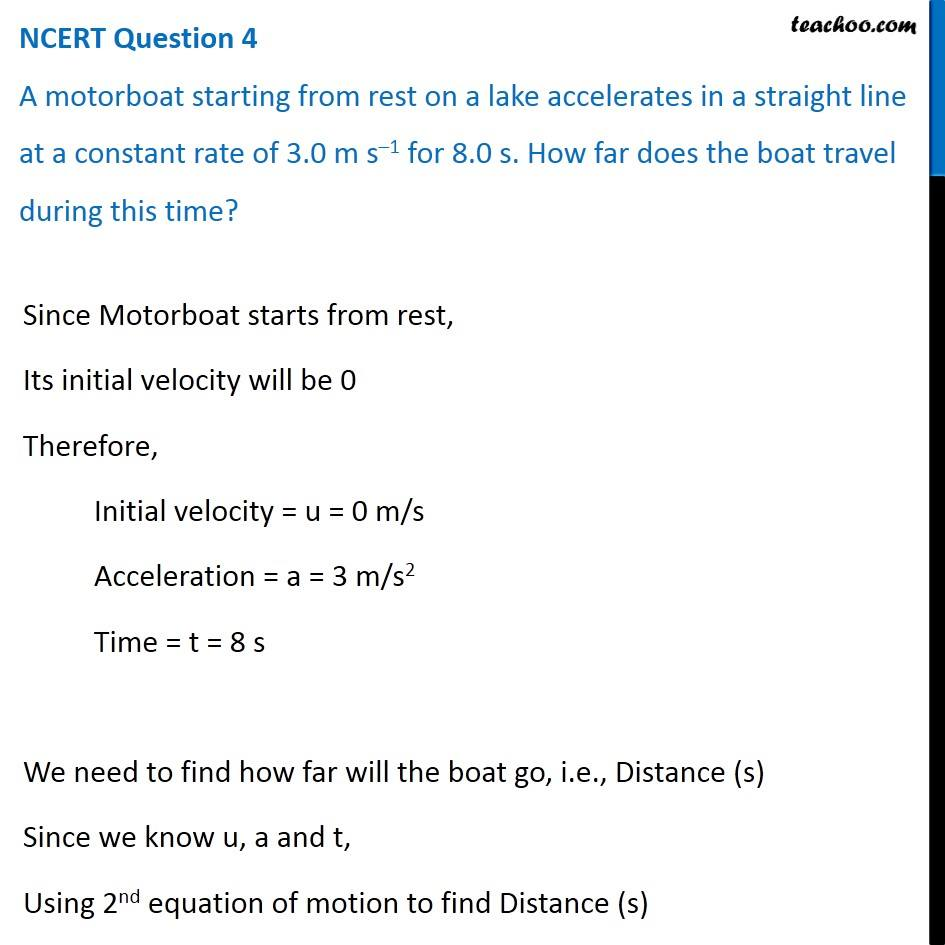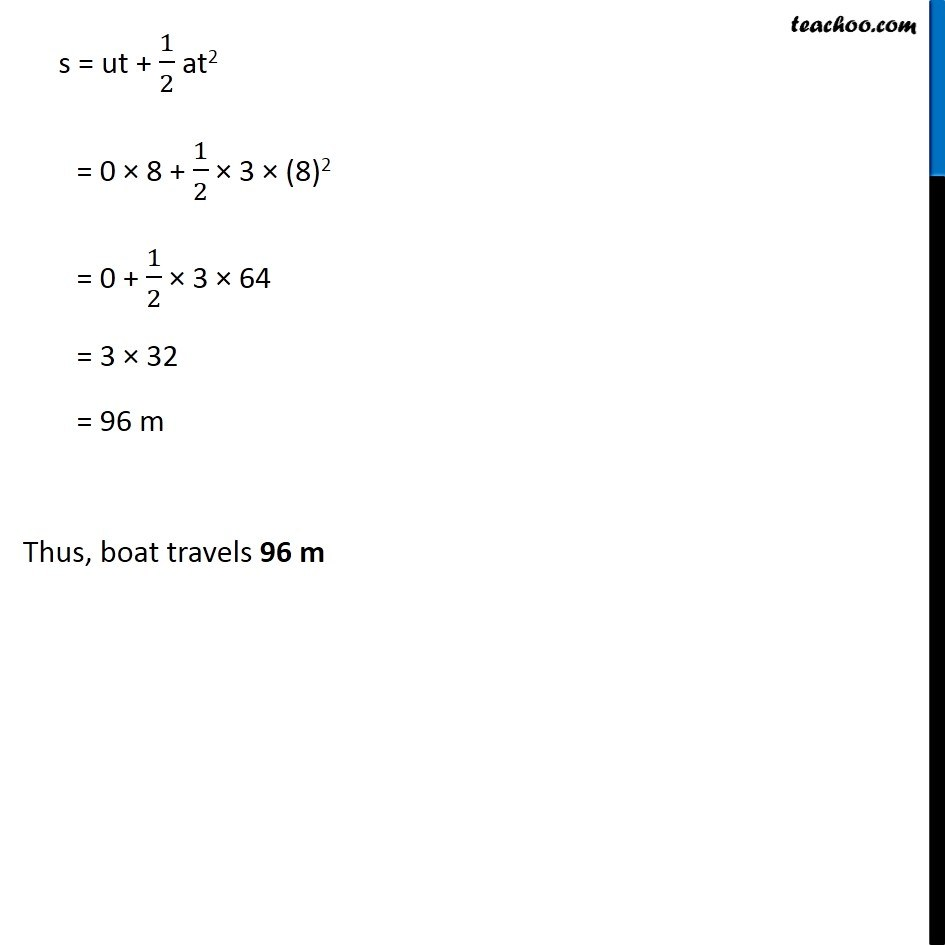NCERT Questions

Class 9
Chapter 8 Class 9 - MotionGet live Maths 1-on-1 Classs - Class 6 to 12

### Transcript

NCERT Question 4 A motorboat starting from rest on a lake accelerates in a straight line at a constant rate of 3.0 m s–1 for 8.0 s. How far does the boat travel during this time? Since Motorboat starts from rest, Its initial velocity will be 0 Therefore, Initial velocity = u = 0 m/s Acceleration = a = 3 m/s2 Time = t = 8 s We need to find how far will the boat go, i.e., Distance (s) Since we know u, a and t, Using 2nd equation of motion to find Distance (s) s = ut + 1/2 at2 = 0 × 8 + 1/2 × 3 × (8)2 = 0 + 1/2 × 3 × 64 = 3 × 32 = 96 m Thus, boat travels 96 m